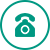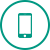### *实验室*

Lab & Experiment

#### Statistical comparisons

• Paired or unpaired t tests. Reports P values and confidence intervals.
• Nonparametric Mann-Whitney test, including confidence interval of difference of medians.
• Kolmogorov-Smirnov test.
• Wilcoxon test with confidence interval of median.
• Perform many t tests at once, using False Discover Rate to choose which comparisons are discoveries to study further.
• Ordinary or repeated measures one-way ANOVA followed by the Tukey, Newman-Keuls, Dunnett, Bonferroni or Holm-Sidak multiple comparison tests, the post-test for trend, or Fisher’s Least Significant tests.
• Many multiple comparisons test are accompanied by confidence intervals and multiplicity adjusted P values.
• Greenhouse-Geisser correction so repeated measures one-way ANOVA does not have to assume sphericity. When this is chosen, multiple comparison tests also do not assume sphericity.
• Kruskal-Wallis or Friedman nonparametric one-way ANOVA with Dunn's post test.
• Fisher's exact test or the chi-square test. Calculate the relative risk and odds ratio with confidence intervals.
• Two-way ANOVA, even with missing values with some post tests.
• Two-way ANOVA, with repeated measures in one or both factors. Tukey, Newman-Keuls, Dunnett, Bonferron, Holm-Sidak, or Fishers LSD multiple comparisons testing main and simple effects.
• Kaplan-Meier survival analysis. Compare curves with the log-rank test (including test for trend).

#### Column statistics

• Calculate min, max, quartiles, mean, SD, SEM, CI, CV,
• Mean or geometric mean with confidence intervals.
• Frequency distributions (bin to histogram), including cumulative histograms.
• Normality testing by three methods.
• One sample t test or Wilcoxon test to compare the column mean (or median) with a theoretical value.
• Skewness and Kurtosis.
• Identify outliers using Grubbs or ROUT method.

#### Linear regression and correlation

• Calculate slope and intercept with confidence intervals.
• Force the regression line through a specified point.
• Fit to replicate Y values or mean Y.
• Test for departure from linearity with a runs test.
• Calculate and graph residuals.
• Compare slopes and intercepts of two or more regression lines.
• Interpolate new points along the standard curve.
• Pearson or Spearman (nonparametric) correlation.

#### Nonlinear regression

• Fit one of our 105 built-in equations, or enter your own.
• Enter differential or implicit equations.
• Enter different equations for different data sets.
• Global nonlinear regression – share parameters between data sets.
• Robust nonlinear regression.
• Automatic outlier identification or elimination.
• Compare models using extra sum-of-squares F test or AICc.
• Compare parameters between data sets.
• Apply constraints.
• Differentially weight points by several methods.
• Accept automatic initial estimated values or enter your own.
• Automatically graph curve over specified range of X values.
• Quantify precision of fits with SE or CI of parameters.
• Quantify symmetry of inprecision with Hougaard’s skewness.
• Plot confidence or prediction bands.
• Test normality of residuals.
• Runs or replicates test of adequacy of model.
• Report the covariance matrix or set of dependencies.
• Easily interpolate points from the best fit curve.

#### Clinical (diagnostic) lab statistics

• Bland-Altman plots.
• Receiver operator characteristic (ROC) curves.
• Deming regression (type ll linear regression).

#### System requirements

• Prism 6 for Windows runs under Windows 2000, XP, Vista, Windows 7 and Windows 8. It runs on both 32- and 64-bit versions. It doesn't matter whether which edition of Windows (Home, Professional, Ultimate)  you use. Prism 6 does not run under Windows 3.1, 95, 98 or Windows NT. Prism will run in a screen as small as 800 x 576. Prism runs fine as a desktop app under Windows 8, but is not a Metro app so will not run on Windows 8 RT (tablet) computers.
• Prism 6 for Macintosh is a Universal application that runs on Intel – and PowerPC-based Mac computers using OS X 10.4 (Tiger), 10.5 (Leopard), 10.6 (Snow Leopard), 10.7 (Lion), 10.8 (Mountain Lion), and 10.9 (Mavericks).
• Prism requires 90 megabytes of space on the hard drive.微信扫码 关注我们

•24小时咨询热线

•移动电话